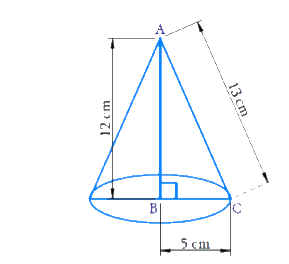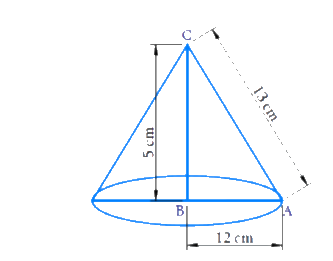# Exercise E13.7 Surface Areas and Volumes NCERT Solutions Class 9

Go back to  'Surface Areas and Volumes'

## Chapter 13 Ex.13.7 Question 1

Assume \begin{align}\pi = \frac{{22}}{7} \end{align} unless stated otherwise

Find the volume of the right circular cone with

(i) radius $$6\rm\, cm,$$ height $$7\rm\, cm$$

(ii) radius $$3.5\rm\, cm,$$ height $$12\rm\, cm$$

### Solution

Reasoning:

Volume of the cone \begin{align} = \frac{1}{3}\pi {r^2}h \end{align}

Where $$r$$ is base radius and $$h$$ is height.

What is known?

Radius and height of two cones.

What is unknown?

Volume of the cone.

Steps:

Radius $$(r) = 6\rm\, cm$$

Height $$(h) = 7\rm\, cm$$

Volume of cone
\begin{align} & = \frac{1}{3}\pi {r^2}h \\ &= \frac{1}{3} \times \frac{{22}}{7} \times 6 \times 6 \times 7\\ &= \,\,264\,\,\rm c{m^3} \end{align}

Radius $$(r) = 3.5\rm\, cm$$

Height $$(h) = 12\rm\, cm$$

Volume of the cone
\begin{align}&= \frac{1}{3}\pi {r^2}h \\ &= \frac{1}{3} \times \frac{{22}}{7} \times 3.5 \times 3.5 \times 12\\ &= 154\,\,\rm c{m^3} \end{align}

Answer:

Volume of the cone \begin{align} = 264\,\,\rm c{m^3} \end{align}

Volume of the cone \begin{align} = 154\,\,\rm c{m^3} \end{align}

## Chapter 13 Ex.13.7 Question 2

Find the capacity in litres of a conical vessel with

(i) radius $$7\rm\, cm,$$ slant height $$25\rm\, cm$$

(ii) height $$12\rm\, cm,$$ slant height $$13\rm\, cm$$

### Solution

Reasoning:

Capacity of a conical vessel is nothing but the Volume of the cone \begin{align} = \frac{1}{3}\pi {r^2}h \end{align}

What is  known?

Radius $$(r) = 7\rm\, cm$$

Slant height $$(1) = 25\rm\, cm$$

What is  unknown?

Capacity of the vessel in litres.

Steps:

Capacity of the conical vessel $$=$$ volume of cone \begin{align} = \frac{1}{3}\pi {r^2}h \end{align}

Where \begin{align}h = \sqrt {{l^2} - {r^2}} \end{align}

Radius \begin{align}(r) = 7\rm \,\rm\,cm \end{align}

Slant height \begin{align}(l) = 25\rm\,\rm \,cm \end{align}

\begin{align}\therefore h &= \sqrt {{{(25)}^2} - {7^2}} \\ &= \sqrt {(25 + 7)(25 - 7)}\\ {\rm{ [ (}}{{\rm{a}}^2} - {b^2}) &= (a + b)(a - b)]\\ &= \sqrt {32 \times 18} \\ &=\! \sqrt {2 \!\times\! 2\! \times\! 2\! \times \!2\! \times \!2 \!\times\! 3 \!\times \!3\! \times\! 2} \\ &= \sqrt {576} \\ &= 24\,\,\rm\,cm \end{align}

Volume
\begin{align}& = \frac{1}{3} \times \frac{{22}}{7} \times 7 \times 7 \times 24\\ &= 1232\,\,\rm\,c{m^3}\,\,or\,\,1.232\,\text{l} \end{align}

\begin{align} [\therefore 1000\,\,c{m^3} = 1\,l] \end{align}

What is  known?

Radius $$(r) = 12 \rm\,cm$$

Slant height \begin{align}(l) = 13\,\,\rm\,cm \end{align}

What is  unknown?

Capacity of the vessel in litres.

Steps:

Capacity of the conical vessel $$=$$ volume of cone \begin{align} = \frac{1}{3}\pi {r^2}h \end{align}

Height $$(h) = 12\rm\, cm$$

Slant height \begin{align}(l) = 13\,\,\rm\,cm \end{align}

\begin{align}&{r^2} + {h^2} = {l^2} \\ &{r^2} = {l^2} - {h^2} \\ &r=\sqrt{l^{2}-h^{2}} \\ &= \sqrt{(13)^{2}-(12)^{2}} \\ &=\sqrt{(13+12)(13-12)} \\&= \sqrt {25} \\ &= 5\,\,\rm\,cm \end{align}

Capacity

\begin{align}&= \frac{1}{3} \times \frac{{22}}{7} \times 5 \times 5 \times 12\\&= \frac{{2200}}{7}\\& = \frac{2200}{7000}\\& = \frac{11}{35}l \end{align}

Answer:

Capacity $$=1.232\,\, l$$

Capacity \begin{align}=\frac{{11}}{{35}} \,l \end{align}

## Chapter 13 Ex.13.7 Question 3

The height of a cone is $$15\rm\, cm.$$ If its volume is \begin{align}1570\,\,c{m^3} \end{align}, find the radius of the base.(Use $$p = 3.14$$).

### Solution

Reasoning:

Volume of the cone is \begin{align} \frac{1}{3} \end{align} times of the volume of a cylinder having radius r and height h i.e. \begin{align} = \frac{1}{3}\pi {r^2}h \end{align}

What is  known?

Volume of the cone and the height of the cone.

What is  unknown?

Radius of the base.

Steps:

Height $$(h) = 15\,\rm cm$$

Radius $$(r) = ?$$

Volume of cone

\begin{align}&= \frac{1}{3}\pi {r^2}h \\1570&= \frac{1}{3}\pi {r^2}h \\ 1570 &= \frac{1}{3} \times 3.14 \times {r^2} \times 15 \\{r^2} &= \frac{{1570 \times 3}}{{15 \times 3.14}} = \frac{{4710}}{{47.1}} \\{r^2} &= 100 \\ r &= \sqrt {100} = 10\,\,\rm\,cm \end{align}

Answer:

Radius of the base $$= 10 \rm\,cm$$

## Chapter 13 Ex.13.7 Question 4

If the volume of a right circular cone of height $$9\rm\, cm$$ is \begin{align}48\pi \,\,\rm\,c{m^3} \end{align}, find the diameter of its base.

### Solution

Reasoning:

Volume of the cone is \begin{align}\frac{1}{3}\end{align} time of the volume of a cylinder having radius $$r$$ and height $$h$$ \begin{align}\frac{1}{3}\pi {r^2}h \end{align}

What is known?

Volume and height of the cone.

What is unknown?

Base diameter.

Steps:

Height $$(h) = 9 \rm\,cm$$

\begin{align} \text{Volume of cone} &= \frac{1}{3}\pi {r^2}h \\48\pi &= \frac{1}{3}\pi {r^2}h \\48\pi &= \frac{1}{3}\pi \times {r^2} \times 9 \\{r^2} &= \frac{{48 \times 3}}{{3 \times 3}} \\&= 16\\r &= \sqrt {16} \\&= 4\,\,\rm\,cm \end{align}

Base diameter \begin{align} = 2\,r = 2 \times 4 = 8\,\,\rm\,cm \end{align}

Answer:

The diameter of the box of the night circular cone is $$8\rm\, cm.$$

## Chapter 13 Ex.13.7 Question 5

A conical pit of top diameter $$3.5\rm\, m$$ is $$12\rm\, m$$ deep. What is its capacity in kiloliters?

### Solution

Reasoning:

Volume of the cone is \begin{align}\frac{1}{3} \end{align}times of the volume of a cylinder having radius r and height h \begin{align} = \frac{1}{3}\pi {r^2}h \end{align}.

What is  known?

On a meter and the depth of the cone.

What is  unknown?

Volume in kilometer.

Steps:

Volume of cone \begin{align} = \frac{1}{3}\pi {r^2}h \end{align}

Height of the cone $$(h) =$$ depth of the pit.

Height $$(h) = 12\rm\, cm$$

Diameter $$= 2r = 3.5\rm\, m$$

\begin{align}\therefore r = \frac{{3.5}}{2} \end{align}

Volume

\begin{align} = \frac{1}{3} \times \frac{{22}}{7} \times {(1.75)^2} \times 12 = 38.5\,\,\rm\,c{m^3} \end{align}

\begin{align}1\,\,\,\rm\,c{m^3} &= 1000\,\,l = 1\,\,k\,\,l \\\therefore\,\, 38.5\,\,\rm\,c{m^3} &= 38500\,\,l = 38.5\,\,kl \end{align}

Answer:

Capacity in kilolitre \begin{align} = 38.5\,\,kl \end{align}.

## Chapter 13 Ex.13.7 Question 6

The volume of a right circular cone is $$9856$$\begin{align}c{m^3} \end{align}. If the diameter of the base is $$28\rm\, cm,$$ find

(i) height of the cone

(ii) slant height of the cone

(iii) curved surface area of the cone

### Solution

Reasoning:

Volume of the cone is \begin{align}\frac{1}{3} \end{align}times of the volume of a cylinder having radius $$r$$ and height $$h$$\begin{align} = \frac{1}{3}\pi {r^2}h \end{align}.Curved surface area of the cone is \begin{align}\pi rl \end{align}

What is  known?

Volume of the right circular cone and diameter of the base.

i.

What is unknown?

Height of the cone.

Steps:

Radius $$(r) = 28/2 \rm\,cm$$

Volume of cone

\begin{align} &= \frac{1}{3}\pi {r^2}h \\9856 &= \frac{1}{3} \times \frac{{22}}{7} \times \frac{{28}}{2} \times \frac{{28}}{2} \times h \\h &= \frac{{9856 \times 3 \times 7 \times 2 \times 2}}{{22 \times 28 \times 28}} \\&= 48\,\,\rm\,cm \end{align}

ii.

What is  unknown?

Slant height of the cone.

Steps:

Radius $$(r) = 14 \rm\,cm$$

Height $$(h) = 48 \rm\,cm$$

\begin{align}l &= \sqrt {{r^2} + {h^2}} \\ &= \sqrt {{{14}^2} + {{48}^2}} \\ &= \sqrt {196 + 2304} \\ &= \sqrt {2500} \\ &= 50\,\,\,\rm\,cm \end{align}

iii.

What is unknown?

Curved surface area of the cone.

Steps:

Radius $$(r) = 14 \rm\,cm$$

Slant height $$(l) = 50\rm\, cm$$

Curved surface area \begin{align}\, = \pi rl \end{align}

\begin{align} &= \frac{{22}}{7} \times 14 \times 50 \\ &= 2200\,\,\rm\,c{m^2} \end{align}

Answer:

Height of the cone $$= 48\rm\,cm$$

Slant height of the cone $$= 50\rm\,cm$$

Surface area\begin{align}\, = 2200\,\,\rm\,c{m^2} \end{align}

## Chapter 13 Ex.13.7 Question 7

A right triangle $$ABC$$ with sides $$5\rm\, cm,$$ $$12 \rm\,cm$$ and $$13\rm\, cm$$ is revolved about the side $$12 \rm\,cm.$$ Find the volume of the solid so obtained.

### Solution

Reasoning:

Volume of the cone is \begin{align}\frac{1}{3} \end{align} times of the volume of a cylinder \begin{align} = \frac{1}{3}\pi {r^2}h \end{align}.

What is known?

Sides of the right triangle.

What is unknown?

Volume of the solid obtained.

Steps:Radius $$(r) = 5\rm\, cm$$

height $$(h) = 12 \rm\,cm$$

Volume of cone

\begin{align}&= \frac{1}{3}\pi {r^2}h \\ &= \frac{1}{3} \times \frac{{22}}{7} \times 5 \times 5 \times 12\\ &= \frac{{2200}}{7}\,\,or\,\,100\pi \end{align}

Answer:

Volume of the cone \begin{align}100\pi \,\,\rm\,c{m^3} \end{align}.

## Chapter 13 Ex.13.7 Question 8

If the triangle $$ABC$$ in the Question 7 above is revolved about the side $$5 \rm\,rm$$ then find the volume of the solid so obtained. Find also the ratio of the volumes of the two solids obtained in Questions 7 and 8.

### Solution

Reasoning:

Volume of the right circular cone is \begin{align}\frac{1}{3} \end{align}time of the volume of a cylinder\begin{align} = \frac{1}{3}\pi {r^2}h \end{align}.

What is  known?

Sides of the right triangle.

What is  unknown?

Volume of the cone and ratio between the volumes.

Solution:Radius of the cone $$= 12\rm\, cm$$

Height of the cone $$= 5 \rm\,cm$$

Volume of cone

\begin{align}&= \frac{1}{3}\pi {r^2}h \\ &= \frac{1}{3} \times \pi \times {(12)^2} \times 5 \\ & = 240\pi \,\,\,\rm\,c{m^3} \end{align}

Volume in question $$7$$\begin{align} = 100\pi \,\,\rm\,c{m^3} \end{align}

Ratio \begin{align}100:240 = 5:12 \end{align}

Answer:

Volume of the cone is \begin{align} = 240\pi \,\,\rm\,c{m^3} \end{align}

Ratio between the volume \begin{align}=5:12 \end{align}

## Chapter 13 Ex.13.7 Question 9

A heap of wheat is in the form of a cone whose diameter is $$10.5\rm\,m$$ and height is $$3\rm\, m.$$ Find its volume. The heap is to be covered by canvas to protect it from rain. Find the area of the canvas required.

### Solution

Reasoning:

Volume of the right circular cone is \begin{align}\frac{1}{3} \end{align}times of the volume of a cylinder. Curved surface area of the cone =\begin{align}\pi rl \end{align}

What is  known?

Diameter and height of the cone.

What is  unknown?

Volume of the cone and area of the canvas to cover the heap.

Steps:

Diameter $$= 2r = 10.5 \rm\,m$$

\begin{align}r = \frac{{10.5}}{2} = 5.25m \end{align}

Height $$(h) = 3 \rm\,m$$

Volume of cone

\begin{align}&= \frac{1}{3}\pi {r^2}h \\ &= \frac{1}{3} \times \frac{{22}}{7} \times {(\frac{{10.5}}{2})^2} \times 3 \\ &= 86.625\,\,\,\rm\,{m^3} \end{align}

Slant height

\begin{align} &= \sqrt {{r^2} + {h^2}} \\&= \sqrt {{{(5.25)}^2} + {{(3)}^2}} \\ &= \sqrt {27.5625 + 9} \\ &= \sqrt {36.5625}\\ &= 6.05\,\,\rm\,m \end{align}

The area of the canvas to cover the heap of wheat $$=$$ surface area of the cone $$=$$ \begin{align}\pi rl \end{align}

\begin{align}& = \frac{{22}}{7} \times \frac{{10.5}}{2} \times 6.05 \\ &= 99.825\,\,\rm\,{m^2} \end{align}

Answer:

Volume of the cone \begin{align} = 86.625\,\,\rm\,{m^3} \end{align}

Area of the canvas required \begin{align} = 99.825\,\,\rm\,{m^2} \end{align}

Related Sections
Related Sections
Instant doubt clearing with Cuemath Advanced Math Program